Turkish Journal of Analysis and Number Theory
ISSN (Print): 2333-1100 ISSN (Online): 2333-1232
Journal Browser
Article Metrics
• 646
Views
• 573
Saves
• 0
Citations
• 0
Likes
Export Article
Turkish Journal of Analysis and Number Theory. 2020, 8(2), 39-48
DOI: 10.12691/tjant-8-2-4
Open AccessArticle

### Mean Values of Arithmetic Functions under Congruences with the Euler Function

1Montanuniversität Leoben Department Mathematik und Informationstechnologie, Austria

2Department of Mathematics, Faculty of Sciences of Monastir, Tunisia

Pub. Date: July 13, 2020

Cite this paper:
Myriam Amri and Khadija Mbarki. Mean Values of Arithmetic Functions under Congruences with the Euler Function. Turkish Journal of Analysis and Number Theory. 2020; 8(2):39-48. doi: 10.12691/tjant-8-2-4

### Abstract

We examine the average order of some arithmetic functions written as sums over Euler function in arithmetic progression and in general over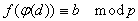such that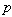is a prime number,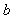an integer and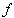is a polynomial function with integer coefficients and a degree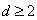that is not constant moduloOur results are based on various estimates of rational exponential sums with the Euler Function in arithmetic progression which are due to William Banks and Igor E. Shparlinski.

Keywords:
arithmetic functions exponential sumsThis work is licensed under a Creative Commons Attribution 4.0 International License. To view a copy of this license, visit http://creativecommons.org/licenses/by/4.0/

### References:

  Apostol T. M, Introduction to analytic number theory, Undergraduate texts in Mathematics New-York Heidelberg-Verlag, 1976.  Banks and Shparlinski I, “Congruences and Rational Exponential Sums with the Euler Function”, Rocky Mountain Journal of Mathematics, October 2006.  Banks and Shparlinski, “Congruences and exponential sums with the sum of aliquot divisors function”, International journal of Number Theory, 2008.  Finsch S.R., “Mathematical constants”, Cambridge University Press, 135-145, 2003.  Kerr B, “Rational exponential sums over the divisor function”, available: arXiv: 1309. 6021, 2013.  Kinkelin H., “Über eine mit der Gammafunktion verwandte Transcendente und deren Anwendung auf die Integralrechnung”, J.Reine Angew. Math, 57, 122-158, 1860.  L. G. Sathe, “On a congruence property of the divisor function”, Am. J. Math., Vol. 67, 397-406, 1945.  Shparlinski, Igor E., “Open problems on exponential and character sums”, Series on Number Theory and Its ApplicationsNumber Theory, Vol. 6, 222-242, 2009.